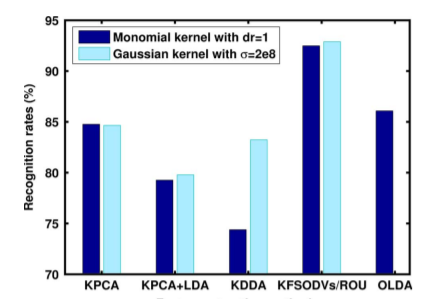# A Rank-One Update Algorithm for Fast Solving Kernel Foley-Sammon Optimal Discriminant Vectors### Abstract

Discriminant analysis plays an important role in statistical pattern recognition. A popular method is the Foley–Sammon optimal discriminant vectors (FSODVs) method, which aims to find an optimal set of discriminant vectors that maximize the Fisher discriminant criterion under the orthogonal constraint. The FSODVs method outperforms the classic Fisher linear discriminant analysis (FLDA) method in the sense that it can solve more discriminant vectors for recognition. Kernel Foley–Sammon optimal discriminant vectors (KFSODVs) is a nonlinear extension of FSODVs via the kernel trick. However, the current KFSODVs algorithm may suffer from the heavy computation problem since it involves computing the inverse of matrices when solving each discriminant vector, resulting in a cubic complexity for each discriminant vector. This is costly when the number of discriminant vectors to be computed is large. In this paper, we propose a fast algorithm for solving the KFSODVs, which is based on rank-one update (ROU) of the eigensytems. It only requires a square complexity for each discriminant vector. Moreover, we also generalize our method to efficiently solve a family of optimally constrained generalized Rayleigh quotient (OCGRQ) problems which include many existing dimensionality reduction techniques. We conduct extensive experiments on sev- eral real data sets to demonstrate the effectiveness of the proposed algorithms

Type
Publication
Transactions on Neural Networks